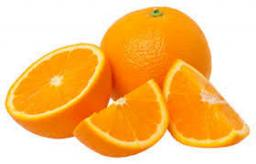# Oranges 5927

When you buy 3 kg of apples and 2 kg of oranges, we will pay € 12. When you buy 2 kg of apples and 4 kg of oranges, we pay € 16. How much does 1 kg of apples cost?

j =  2
p =  3

### Step-by-step explanation:

3j+2p = 12
2j+4p = 16

3·j+2·p = 12
2·j+4·p = 16

3j+2p = 12
2j+4p = 16

Row 2 - 2/3 · Row 1 → Row 2
3j+2p = 12
2.67p = 8

p = 8/2.66666667 = 3
j = 12-2p/3 = 12-2 · 3/3 = 2

j = 2
p = 3

Our linear equations calculator calculates it.Did you find an error or inaccuracy? Feel free to write us. Thank you!

Tips for related online calculators
Do you have a system of equations and looking for calculator system of linear equations?